# NCERT Solutions for Class 8 Maths Exercise 16.1## myCBSEguide App

CBSE, NCERT, JEE Main, NEET-UG, NDA, Exam Papers, Question Bank, NCERT Solutions, Exemplars, Revision Notes, Free Videos, MCQ Tests & more.

NCERT solutions for Class 8 Maths Playing with Numbers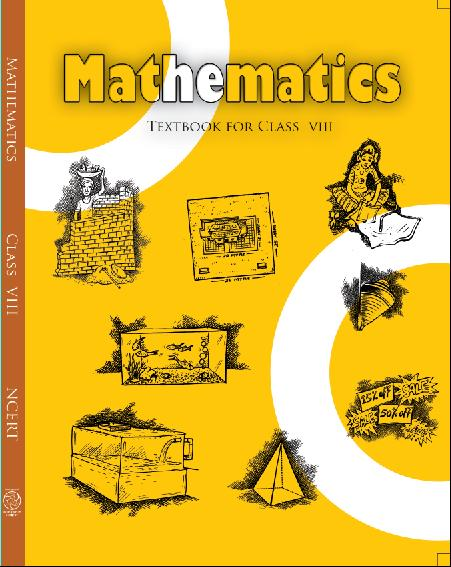## NCERT Solutions for Class 8 Maths Playing with Numbers

##### NCERT SOLUTION
###### Find the values of the letters in each of the following and give reasons for the steps involved.

1.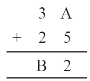Ans. On putting A = 1, 2, 3, 4, 5, 6, 7 and so on and we get, 7 + 5 = 12 in which ones place is 2.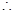A = 7

And putting 2 and carry over 1, we get

B = 6

Hence A = 7 and B = 6

###### 2.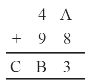Ans. On putting A = 1, 2, 3, 4, 5, 6, 7 and so on and we get,8 + 5 = 13 in which ones place is 3.A = 5

And putting 3 and carry over 1, we get

B = 4 and C = 1

Hence A = 5, B = 4 and C = 1

NCERT Solutions for Class 8 Maths Exercise 16.1

###### 3.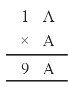Ans.  On putting A = 1, 2, 3, 4, 5, 6, 7 and so on and we get, A x A = 6 x 6 = 36 in which ones place is 6.A = 6

Hence A = 6

NCERT Solutions for Class 8 Maths Exercise 16.1

###### 4.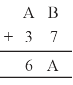Ans. Here, we observe that B = 5

so that 7 + 5 = 12.

Putting 2 at ones place and carry over 1 and A = 2, we get

2 + 3 + 1 = 6

Hence A = 2 and B = 5

###### 5.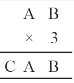Ans. Here on putting B = 0,

we get 0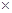3 = 0.

And A = 5, then 53 = 15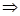A = 5 and C = 1

Hence A = 5, B = 0 and C = 1

NCERT Solutions for Class 8 Maths Exercise 16.1

###### 6.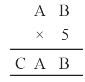Ans. On putting B = 0, we get 0 , 5 = 0 and A = 5, then 55 = 25A = 5, C = 2

Hence A = 5, B = 0 and C = 2

NCERT Solutions for Class 8 Maths Exercise 16.1

###### 7.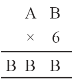Ans.  Here product of B and 6 must be same as ones place digit as B.

61 = 6, 62 = 12, 63 = 18,

64 = 24

On putting B = 4, we get the ones digit 4 and remaining two B’s value should be 44.For 67 = 42 + 2 = 44

Hence A = 7 and B = 4

###### 8.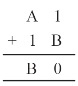Ans. On putting B = 9, we get 9 + 1 = 10

Putting 0 at ones place and carry over 1, we get

For A = 77 + 1 + 1 = 9

Hence A = 7 and B = 9

NCERT Solutions for Class 8 Maths Exercise 16.1

###### 9.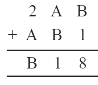Ans. On putting B = 7,7 + 1 = 8

Now A = 4, then 4 + 7 = 11

Putting 1 at tens place and carry over 1, we get

2 + 4 + 1 = 7

Hence A = 4 and B = 7

NCERT Solutions for Class 8 Maths Exercise 16.1

###### 10.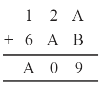Ans. Putting A = 8 and B = 1, we get

8 + 1 = 9

Now again we add 2 + 8 = 10

Tens place digit is ‘0’ and carry over 1.

Now 1 + 6 + 1 = 8 = A

Hence A = 8 and B = 1

## NCERT Solutions for Class 8 Maths Exercise 16.1

NCERT Solutions Class 8 Mathematics PDF (Download) Free from myCBSEguide app and myCBSEguide website. Ncert solution class 8 Mathematics includes text book solutions from Class 8 Maths Book . NCERT Solutions for CBSE Class 8 Maths have total 16 chapters. 8 Maths NCERT Solutions in PDF for free Download on our website. Ncert class 8 solutions PDF and Maths ncert class 8 PDF solutions with latest modifications and as per the latest CBSE syllabus are only available in myCBSEguide.

## CBSE app for Class 8

To download NCERT Solutions for class 8 Social Science, Computer Science, Home Science,Hindi ,English, Maths Science do check myCBSEguide app or website. myCBSEguide provides sample papers with solution, test papers for chapter-wise practice, NCERT solutions, NCERT Exemplar solutions, quick revision notes for ready reference, CBSE guess papers and CBSE important question papers. Sample Paper all are made available through the best app for CBSE students and myCBSEguide website.1. I love this

2. nice